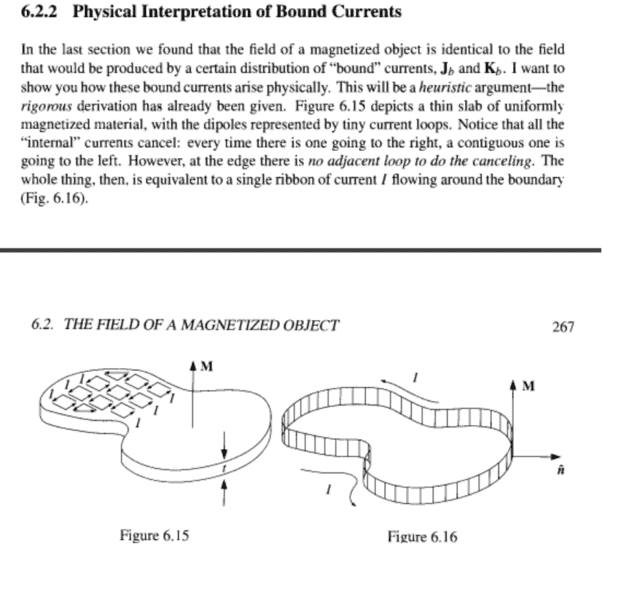# Bound current of a magnetized object

Clara ChungWhy is the current of the boundary uniform?

#### Attachments

Homework Helper
Gold Member
Bound magnetic surface current per unit length ## \vec{K}_m=\vec{M} \times \hat{n} ##. In this case bound magnetic current ## I_m=K_m \, t ##. ## \\ ## (Defining ## M ## such that ## B=\mu_o(H+M) ##, as opposed to ## B=\mu_oH+M ##). ## \\ ## For uniform ## M ##, you compute ## K_m=M \times \hat{n} ## at all surfaces. If ## M ## is parallel to ## \hat{n} ##, then ## K_m=0 ## at that point. This concept of magnetic surface currents becomes very useful in explaining how the magnetic field arises from a cylindrical permanent magnet that has uniform magnetization ## \vec{M} ## along the axis. For the magnetized cylinder, the surface currents are like the current that flows on a solenoid. They are geometrically equivalent. Biot-Savart and/or Ampere's law can be used to compute the magnetic field ## B ## from the surface currents of the permanent magnet, both outside and inside the magnet. ## \\ ## In general, bound magnetic current density is ## J_m=\nabla \times \vec{M } ##. With Stokes' theorem at a boundary where ## \vec{M} ## is discontinuous, this becomes surface current per unit length ## K_m=\vec{M} \times \hat{n} ##. ## \\ ## See also: https://www.physicsforums.com/insights/permanent-magnets-ferromagnetism-magnetic-surface-currents/ This Insights uses cgs units, but hopefully it is still readable.

Last edited:
•Clara Chung
Clara Chung
Bound magnetic surface current per unit length ## \vec{K}_m=\vec{M} \times \hat{n} ##. In this case bound magnetic current ## I_m=K_m \, t ##. ## \\ ## (Defining ## M ## such that ## B=\mu_o(H+M) ##, as opposed to ## B=\mu_oH+M ##). ## \\ ## For uniform ## M ##, you compute ## K_m=M \times \hat{n} ## at all surfaces. If ## M ## is parallel to ## \hat{n} ##, then ## K_m=0 ## at that point. This concept of magnetic surface currents becomes very useful in explaining how the magnetic field arises from a cylindrical permanent magnet that has uniform magnetization ## \vec{M} ## along the axis. For the magnetized cylinder, the surface currents are like the current that flows on a solenoid. They are geometrically equivalent. ## \\ ## In general, bound magnetic current density is ## J_m=\nabla \times \vec{M } ##. With Stokes' theorem at a boundary where ## \vec{M} ## is discontinuous, this becomes surface current per unit length ## K_m=\vec{M} \times \hat{n} ##. ## \\ ## See also: https://www.physicsforums.com/insights/permanent-magnets-ferromagnetism-magnetic-surface-currents/ This Insights uses cgs units, but hopefully it is still readable.
Thank you for the answer. However, I don't understand the physical explanation of bound current. In the picture, current of magnetic dipoles are canceled in the interior of the object and the current of each dipoles in the edge contributes to the current flowing around the boundary. I don't know why this current flowing around the boundary is considered as a constant quantity, what is the physical reason behind?

Homework Helper
Gold Member
Consider a checkerboard, where on each square of the checkerboard, a current flows ## I ## in a clockwise direction around each individual square. Currents from adjacent squares will cancel, and the net result will be a current ## I ## flowing in the clockwise direction around the outside of the checkerboard. ## \\ ## This is basically how the magnetization currents from the individual atoms sums in the case of uniform magnetization ## \vec{M} ##. Adjacent currents cancel when the magnetization is uniform, but edge/surface effects appear.

•Clara Chung
Homework Helper
Gold Member
Current can't "build up" anywhere along the edge so current must be constant everywhere along the edge. Same as Kirchhoff.

•Clara Chung